International
Tables for
Crystallography
Volume A
Space-group symmetry
Edited by Th. Hahn

International Tables for Crystallography (2006). Vol. A, ch. 14.2, pp. 871-872

Section 14.2.3.3. Lattice complexes with degrees of freedom

W. Fischera and E. Kocha*

aInstitut für Mineralogie, Petrologie und Kristallographie, Philipps-Universität, D-35032 Marburg, Germany
Correspondence e-mail:  kochelke@mailer.uni-marburg.de

14.2.3.3. Lattice complexes with degrees of freedom

| top | pdf |

The descriptive symbols of lattice complexes with degrees of freedom consist, in general, of four parts: shift vector, distribution symmetry, central part and site-set symbol. Either of the first two parts may be absent.

Example..2 C4xxz is the descriptive symbol of the lattice complex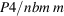in its characteristic position:is the shift vector, ..2 the distribution symmetry, C the central part and 4xxz the site-set symbol.

Normally, the central part is the symbol of an invariant lattice complex. Shift vector and central part together should be interpreted as described in Section 14.2.3.2. The point configurations of the regarded Wyckoff position can be derived from that described by the central part by replacing each point by a finite set of points, the site set. All points of a site set are symmetrically equivalent under the site-symmetry group of the point that they replace. A site set is symbolized by a string of numbers and letters. The product of the numbers gives the number of points in the site set, whereas the letters supply information on the pattern formed by these points. Site sets replacing different points may be differently oriented. In this case, the distribution-symmetry part of the reference symbol shows symmetry operations that relate such site sets to one another. The orientation of the corresponding symmetry elements is indicated as in the oriented site-symmetry symbols (cf. Section 2.2.12). If all site sets have the same orientation, no distribution symmetry is given.

Examples

 (1)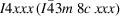designates a lattice complex, the point configurations of which are composed of tetrahedra 4xxx in parallel orientations replacing the points of a cubic body-centred lattice I. The vertices of these tetrahedra are located on body diagonals. (2)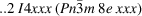represents the lattice complex for which, in contrast to the first example, the tetrahedra 4xxx around 000 and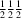differ in their orientation. They are related by a twofold rotation ..2 . (3)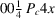is the descriptive symbol of Wyckoff position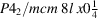. Each corresponding point configuration consists of squares of points 4x replacing the points of a tetragonal primitive lattice P. In comparison with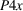,shows a unit-cell enlargement by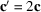and a subsequent shift by the vector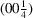.

In the case of a Weissenberg complex, the central part of the descriptive symbol always consists of two (or more) symbols of invariant lattice complexes belonging to the same crystal family and forming limiting complexes of the regarded Weissenberg complex. The shift vector then refers to the first limiting complex. The corresponding site-set symbols are distinguished by containing the number 1 as the only number, i.e. each site set consists of only one point.

Example

In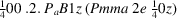, each of the two points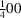and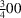, represented by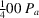, is replaced by a site set containing only one point 1z, i.e. the points are shifted along the z axis. The shifts of the two points are related by a twofold rotation .2., i.e. are running in opposite directions. The point configurations of the two limiting complexes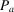and B refer to the special parameter valuesand, respectively.

The central parts of some lattice complexes with two or three degrees of freedom are formed by the descriptive symbol of a univariant Weissenberg complex instead of that of an invariant lattice complex. This is the case only if the corresponding characteristic space-group type does not refer to a suitable invariant lattice complex.

Example

In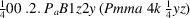, each of the two points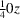and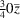, represented by, is replaced by a site set 2y of two points forming a dumb-bell. These dumb-bells are oriented parallel to the y axis.

The symbol of a noncharacteristic Wyckoff position is deduced from that of the characteristic position. The four parts of the descriptive symbol are subjected to the transformation necessary to map the characteristic Wyckoff position onto the Wyckoff position under consideration.

Example

The lattice complex with characteristic Wyckoff position Imma 8h 0yz has the descriptive symbol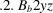for this position. Another Wyckoff position of this lattice complex is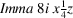. The corresponding point configurations are mapped onto each other by interchanging positive x and negative y directions and shifting by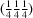. Therefore, the descriptive symbol for Wyckoff position Imma i is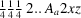.

In some cases, the Wyckoff position described by a lattice-complex symbol has more degrees of freedom than the lattice complex (see Section 14.2.2.1). In such a case, a letter (or a string of letters) in brackets is added to the symbol.

Examples

tP[z] for P4 a, aP[xyz] for P1 a.